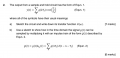# "Sample and Hold" Circuit and Transfer Function

#### marathonman

Joined Mar 4, 2015
4
Hi.

I am trying to answer the following question. It is taken from a past exam paper at my university, and I think there are multiple errors in there. I am not very happy with the lecturer, and I wanted some other opinions as I might just be misunderstanding the topic. Here is the question:Here are my issues:

1) in Equn. 1 he has not defined what g(t) is. I am assuming that it is the input to the sample and hold circuit, but this is a guess. Without this knowledge it seems to me to be impossible to answer part (a).

2) If we assume that g(t) is the input to the circuit, I think Equn. 1 is still incorrect. Surely it should be something like $$y(t)=\sum g(kT_s)rect(\frac{t-kT_s}{T_s})$$ , as you would want a train of rect functions not just a single one?!

3) He gives the answer to part (a) as $$H(\omega)=T_s rect(\frac{\omega}{4\pi B})$$. I don't see how this can be the case, since the Fourier Transform of the rect function is the sinc function, so in the time domain you would be convolving g(t) with the sinc function. How could this give a train of rect functions as its output?

4) There is also another error in Equn. 2 which is pretty obvious.

I would welcome any thoughts and feedback on the issues above.

Thank you!